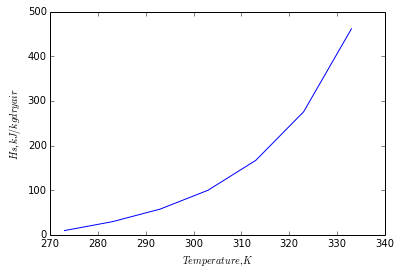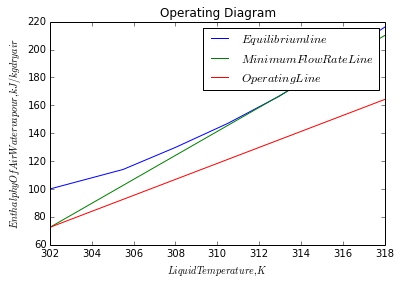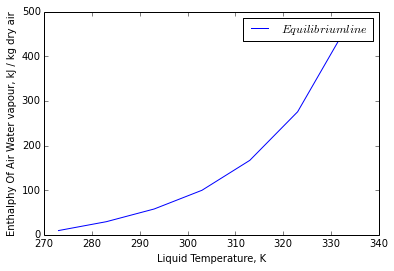# Chapter 8: Humidification Operations¶

### Example 8.1,Page number:479¶

In :
#Variable declaration

P_total = 1  						# [bar]
T1 = 320.0  						# [K]
T_c = 562.2  						# [K]
P_c = 48.9  						# [bar]
A = -6.983
B = 1.332
C = -2.629
D = -3.333
import math
from scipy.optimize import fsolve
from pylab import *

x1 = 1-(T1/T_c)
def f12(P1):
return(math.log(P1/P_c)-(A*x1+B*x1**1.5+C*x1**3+D*x1**6)/(1-x1))
P1 = fsolve(f12,0.01) # [bar]
print"Vapor pressure of benzene at 320 K is",round(P1,2),"bar"

M_benzene = 78.0 # [gram/mole]
print"\nSolution 8.1 (a)"

# Solution (a)
# For nitrogen
M_nitrogen = 28.0  				# [gram/mole]
# From equation 8.2
Y = P1/(P_total - P1)  				#[mole C6H6/ mole N2]
Y_s1 = Y*(M_benzene/M_nitrogen)  		# [gram C6H6/gram N2]

#Result

print"Absolute humidity of mixture of benzene and nitrogen is",round(Y_s1,2)," gram C6H6/gram N2\n\n"

print"\nSolution 8.1 (b)\n"
# Solution (b)
# For carbon dioxide
M_carbondioxide = 44.0  				# [gram/mole]
# From equation 8.2
Y = P1/(P_total - P1)  				#[mole C6H6/ mole C02]
Y_s2 = Y*(M_benzene/M_carbondioxide)  		# [gram C6H6/gram CO2]

#Result

print"\nAbsolute humidity of mixture of benzene and carbon dioxide is",round(Y_s2,3),"gram C6H6/gram CO2\n"

Vapor pressure of benzene at 320 K is 0.32 bar

Solution 8.1 (a)
Absolute humidity of mixture of benzene and nitrogen is 1.31  gram C6H6/gram N2

Solution 8.1 (b)

Absolute humidity of mixture of benzene and carbon dioxide is 0.833 gram C6H6/gram CO2



### Example 8.2,Page number:480¶

In :
#Variable declaration
# A - water vapor    B - air
# REference state is air

T_ref = 273  					# [Reference temperature, K]
T = 303  					# [K]
P_total = 1  					# [atm]
P_A = 4.24  					# [Vapor pressure of water at 303K, kPa]
M_A = 18.0  					# [gram/mole]
M_B = 29.0  					# [gram/mole]
C_A = 1.884  					# [kJ/kg.K]
C_B = 1.005  					# [kJ/kg.K]
lamda = 2502.3  				# [Latent heat of Vaporization at 273K, kJ/kg]

#Calculation

P_total = P_total*101.325  			# [kPa]

# From equation 8.2
Y_s = P_A/(P_total - P_A)*(M_A/M_B)  		#[kg H2O/ kg dry air]

print"Absolute humidity of mixture of water vapor and air is",round(Y_s,3),"kg H2O/kg dry air"
# From equation 8.3
H_s = C_B*(T-T_ref) + Y_s*(C_A*(T-T_ref) + lamda)  	# [kJ/kg dry air]

#Result

print"Enthalpy per unit mass of dry air of a saturated mixture at 303 K and 1 atm is",round(H_s,1),"kJ/kg dry air"
print"\n\nFollowing graph shows the result of similar calculations form 273 to 333 K"
x=[273,283,293,303,313,323,333]
y=[9.48,29.36,57.57,99.75,166.79,275.58,461.50]
a=plot(x,y)
xlabel('$Temperature,K$')
ylabel('$Hs,kJ/kg dry air$')
show(a)

Absolute humidity of mixture of water vapor and air is 0.027 kg H2O/kg dry air
Enthalpy per unit mass of dry air of a saturated mixture at 303 K and 1 atm is 99.5 kJ/kg dry air

Following graph shows the result of similar calculations form 273 to 333 K### Example 8.3,Page number:482¶

In :
#Variable declaration
# A - water vapor   B - air

T = 328  					# [dry bulb temperature, K]
P_total = 1.0  					# [atm]
H = 30.0  					# [relative humidity, %]

P_vapA = 15.73  				# [vapor pressure of water, kPa]
P_total = P_total*101.325  			# [kPa]
M_A = 18.0  					# [gram/mole]
M_B = 29.0  					# [gram/mole]

P_A = (H/100)*P_vapA 				# [partial pressure of A,kPa]

#Calculation

print"Solution 8.3 (a)"
# At dew point partial pressure is equal to vapor pressure
# Using Antonnie equation we can find dew point temperature

print"Dew point temperature is 304.5 K\n"

# From equation 8.1
Y_s = P_A/(P_total-P_A)*(M_A/M_B)

print"Absolute humidity of air-water mixture at 328 K is",round(Y_s,2),"kg H2O/kg dry air\n\n"

print"\n Solution8.3 (b)"

#soluton (b)
T_ref = 273  					# [K]
C_A = 1.884  					# [kJ/kg.K]
C_B = 1.005  					# [kJ/kg.K]
lamda = 2502.3  				# [Latent heat of Vaporization at 273 K, kJ/kg]

# From equation 8.3
H_s = C_B*(T-T_ref) + Y_s*(C_A*(T-T_ref) + lamda)

print"Enthalpy per unit mass of dry air of a saturated mixture relative to 273 K is",round(H_s,1),"kJ/kg dry air"

Solution 8.3 (a)
Dew point temperature is 304.5 K

Absolute humidity of air-water mixture at 328 K is 0.03 kg H2O/kg dry air

Solution8.3 (b)
Enthalpy per unit mass of dry air of a saturated mixture relative to 273 K is 134.3 kJ/kg dry air


### Example 8.4,Page number:483¶

In :
#Variable declaration

# a - water vapor    b - air
T_G1 = 356  						# [K]
P_total = 101.325  					# [kPa]
Y_1 = .03  						# [kg water/kg dry air]

C_pa = 1.884 	 					# [kJ/kg.K]
C_pb = 1.005  						# [kJ/kg.K]

C_s1 = C_pb + Y_1*C_pa 					# [kJ/kg.K]

T_1 = 373.15  						# [K]
T_c = 647.1  						# [K]
M_a = 18.02  						# [gram/mole]
M_b = 28.97  						# [gram/mole]
lamda_1 = 2256  					# [Latent Heat of Vaporizarion at T_1,
#Calculation

import math
from scipy.optimize import fsolve
from pylab import *

def f12(T_as):
return(T_as - T_G1 + ((math.exp(16.3872 - (3885.7/(T_as - 42.98)))/(P_total - (math.exp(16.3872 - (3885.7/(T_as - 42.98))))))*(M_a/M_b) - Y_1)*(lamda_1*((1-T_as/T_c)/(1-T_1/T_c))**.38/C_s1))
T_as = fsolve(f12,310)  # [K]

# Now using equation 8.2

P_a = math.exp(16.3872-(3885.7/(T_as-42.98)))  		# [kPa]
Y_as = P_a/(P_total-P_a)*M_a/M_b  			# [kg water/kg dry air]

#Result
print"Absolute humidity is",round(Y_as,3),"kg water/kg dry air\n"

Adiabatic Saturation Temperature is 313.0 K
Absolute humidity is 0.049 kg water/kg dry air



### Example 8.5,Page number:487¶

In :
#Variable declaration

T_w = 320  						# [K]
T_g = 340  						# [K]
lambda_w = 2413  					# [Latent Heat of Vaporization at 320K, 							kJ/kg]
Y_w1 = 0.073  						# [kg water/kg dry air]

A = 0.95  						# [For air water system,A, kJ/kg.K]

#Calculation

#    here A = hg/ky, psychrometric ratio
#    Air-water mixture is saturated at 320K and 1 atm
#    Using equation 8.15

Y_w2 = Y_w1 - ((T_g-T_w)*A/lambda_w)  			# [kg water/kg dry air]

#Result

print"Absolute humidity of air-water mixture at 340 K and 1 atm is",round(Y_w2,3)," kg water/kg dry air\n "

Absolute humidity of air-water mixture at 340 K and 1 atm is 0.065  kg water/kg dry air



### Example 8.6,Page number:487¶

In :
#Variable declaration
# a - toluene    b - air

T_G1 = 333  						# [K]
P_total = 101.325  					# [kPa]
Y_1 = 0.05  						# [kg vapor/kg dry air]

C_pa = 1.256  						# [kJ/kg.K]
C_pb = 1.005  						# [kJ/kg.K]

C_s1 = C_pb + Y_1*C_pa

T_1 = 383.8  						# [K]
T_c = 591.8  						# [K]
M_a = 92  						# [gram/mole]
M_b = 28.97  						# [gram/mole]

#Calculation
lamda_1 = 33.18*1000/92  				# [Latent heat of vaporization at T_1, 							kJ/kg]
import math
from scipy.optimize import fsolve
# Constants of antoine equation
A = 13.9320
B = 3057  						# [K]
C = -55.52  						# [K]

print "\nSolution 8.6 (a)"

# Solution (a)

def f12(T_as):
return(T_as - T_G1 + ((math.exp(13.9320 - (3057/(T_as - 55.52)))/(P_total - (math.exp(13.9320 - (3057/(T_as - 55.52))))))*(M_a/M_b) - Y_1)*(lamda_1*((1-T_as/T_c)/(1-T_1/T_c))**0.38/C_s1))
T_as = fsolve(f12,273)   				# [K]

# Now using equation 8.2

P_a = math.exp(13.9320-(3057/(T_as-55.52)))  		# [kPa]
Y_as = P_a/(P_total-P_a)*M_a/M_b  			# [kg vapor/kg dry air]

#Result

print"Absolute humidity is",round(Y_as,3),"kg vapor/kg dry air"

print"\nSolution 8.6 (b)"

# Solution (b)

# Thermodynamic properties of mixture of toluene and air
row = 1.06  						# [kg/cubic m]
u = 19.5*10**-6  					# [P]
Pr = 0.7
Dab = 0.1  						#[From Wilke-Lee equation, square cm/s]
Sc = u/(row*Dab*10**-4)

# Using equation 8.16

A_1 = C_s1*(Sc/Pr)**0.567  # [kJ/kg.K]
# here A_1 = hg/ky, psychrometric ratio

# Using equation 8.15
#    T_w = T_G1 - (Y_w-Y_1)*lamda_w/(hg/ky)
#   where lamda_w = lamda_1*((1-T_w/T_c)/(1-T_1/T_c))**.38
#   Y_w = P_a/(P_total-P_a)*M_a/M_b
#   P_a = math.exp(A-B/(T+c))

def f15(T_w):
return(T_w - T_G1 + ((math.exp(13.9320 - (3057/(T_w - 55.52)))/(P_total - (math.exp(13.9320 - (3057/(T_w - 55.52))))))*(M_a/M_b) - Y_1)*(lamda_1*((1-T_w/T_c)/(1-T_1/T_c))**.38/A_1))
T_w = fsolve(f15,273)  # [K]
print"Wet bulb Temperature is",round(T_w)," K\n"

# Now using equation 8.2

P_a = math.exp(13.9320-(3057/(T_w-55.52)))  		# [kPa]
Y_w = P_a/(P_total-P_a)*M_a/M_b  			# [kg vapor/kg dry air]

print"Absolute humidity is",round(Y_w,3),"kg vapor/kg dry air\n"

Solution 8.6 (a)
Adiabatic Saturation Temperature is 300.0 K
Absolute humidity is 0.136 kg vapor/kg dry air

Solution 8.6 (b)
Wet bulb Temperature is 305.0  K

Absolute humidity is 0.177 kg vapor/kg dry air



### Example 8.7,Page number:493¶

In :
L_min = 2.27 # [kg/square m.s]
G_min = 2 # [kg/square m.s]
L2_prime = 15 # [kg/s]
Templ2 = 318 # [K]
Tempg1 = 303 # [Entering air dry bulb, K]
Tempw1 = 297 # [ Entering air wet bulb, K]
Kya = 0.90 # [kg/cubic m.s]

import math
from pylab import *
from scipy.optimize import fsolve
from numpy import*

H1_prime = 72.5 # [kJ/kg dry air]
Y1_prime = 0.0190 # [kg water/kg dry air]
Templ1 = 302 # [K]
Cal = 4.187 # [kJ/kg]

# Equilibrium Data:
# Data  = [Temp.(K),H_star(kJ/kg)]
Data_star =matrix([[302,100],[305.5,114],[308,129.8],[310.5,147],[313,166.8],[315.5,191],[318,216]])

# The operating line for least slope:
H2_star = 210 # [kJ/kg]
Data_minSlope =matrix([[Templ1,H1_prime],[Templ2,H2_star]])
def f14(Gmin):
return(((L2_prime*Cal)/Gmin)-((H2_star-H1_prime)/(Templ2-Templ1)))
Gmin = fsolve(f14,2) # [kg/s]
Gs = 1.5*Gmin # [kg/s]

# For the Operating Line:
def f15(H2):
return(((H2-H1_prime)/(Templ2-Templ1))-((L2_prime*Cal)/Gs))
H2 = fsolve(f15,2) # [kJ/kg dry air]
Data_opline =matrix([[Templ1,H1_prime],[Templ2,H2]])

a1=plot(Data_star[:,0],Data_star[:,1],label='$Equilibrium line$')
a2=plot(Data_minSlope[:,0],Data_minSlope[:,1],label='$Minimum Flow Rate Line$')
a3=plot(Data_opline[:,0],Data_opline[:,1],label='$Operating Line$')
legend(loc='upper right')
title('Operating Diagram')
xlabel("$Liquid Temperature, K$")
ylabel("$Enthalphy Of Air Water vapour, kJ / kg dry air$")
show(a1)
show(a2)
show(a3)

# Tower cross section Area:
Al = L2_prime/L_min # [square m]
Ag = Gs/G_min # [square m]
A = min(Al,Ag) # [square m]
print"Cross sectional is",round(A,2)," square m\n"

# Data from operating line:
# Data1 = [Temp.(K),H_prime(kJ/kg)]
Data1 =matrix([[302,72.5],[305.5,92],[308,106.5],[310.5,121],[313,135.5],[315.5,149.5],[318,164.2]])

# Driving Force:
Data2 = zeros((7,2))
# Data2 = [Temp[K],driving Force]
for i in range(0,7):
Data2[i] = Data1[i,0]
Data2[i,1] = 1/(Data_star[i,1]-Data1[i,1])

# The data for operating line as abcissa is plotted against driving force
Area = 3.28
# From Eqn. 7.54
def f16(Z):
return(Area-(Kya*Z/G_min))
Z = fsolve(f16,2)
print"The height of tower is",round(Z,2)
NtoG = 3.28
HtoG = G_min/Kya # [m]

# Make up water
# Assuming the outlet air is essentially saturated:
Y2_prime = 0.048 # [kg water/kg dry air]
H2 = 164.2  # [kJ/kg dry air]
# This corresponds to an exit-air temperature of 312.8 K

# Approximate rate of evaporation
R = Gs*(Y2_prime-Y1_prime)
print"Rate of evaporation is",round(R,3),"kg/s\n"Cross sectional is 5.48  square m

The height of tower is 7.29
Rate of evaporation is 0.318 kg/s



### Example 8.8,Page number:495¶

In :
print"Solution8.8 (a)"

#Variable declaration
# a - water vapor   b - air

T_L2 = 314  				# [inlet water temperature, K]
T_L1 = 303  				# [outlet water temperature, K]
T_d = 306  				# [dry bulb temperature ,K]
T_w1 = 298  				# [wet bulb temperature, K]
Z = 3 		 			# [packed tower depth, m]
G_x = 3  				# [mass velocity, kg/square m.s]
G_s =2.7  				# [mass velocity, kg/square m.s]

import math
from numpy import *
from pylab import *
T_o = 273  				# [reference temperature, K]
C_al = 4.187  				# [kJ/kg.K]
C_pb = 1.005  				# [kJ/kg.K]
C_pa = 1.884  				# [kJ/kg.K]
P_total = 101.325  			# [kPa]
lamda_0 = 2502.3  			# [kJ/kg]
M_a = 18.02  				# [gram/mole]
M_b = 28.97  				# [gram/mole]

# Equilibrium Data:
# Data  = [Temp.(K),H_eqm(kJ/kg)],[H_eqm - Equilibrium gas enthalpy]
Data_eqm =matrix([[273,9.48],[283,29.36],[293,57.8],[303,99.75],[313,166.79],[323,275.58],[333,461.5]])

a1=plot(Data_eqm[:,0],Data_eqm[:,1],label='$Equilibrium line$')

legend(loc='upper right')
xlabel("Liquid Temperature, K")
ylabel("Enthalphy Of Air Water vapour, kJ / kg dry air")

P_a = math.exp(16.3872-(3885.7/(T_w1-42.98)))  				# [kPa]
Y_m1 = P_a/(P_total-P_a)*(M_a/M_b)  					# [kg water/kg dry air]
H_g1 = C_pb*(T_w1-T_o) + Y_m1*(C_pa*(T_w1-T_o)+lamda_0)  		# [Enthalpy of saturated 									mixture, kJ/kg 									dry air]

# From overall energy balance
H_g2 = H_g1 + G_x*C_al*(T_L2-T_L1)/G_s  				# [Enthalpy of exit air, 									kJ/kg]

# For calculation of mass transfer unit, Ntog
# Data1 = [T_L1 H_g1,.....,T_L2 H_g2]

deltaT = (T_L2-T_L1)/9

# Data for enthalpy of exit air at different temperature varying from T_L1 to T_L2, 			operating line
Data1=matrix([[303,76.17],[304.22,81.85],[305.44,87.53],[306.67,93.22],[307.89,98.91],[309.11,104.59],[310.33,110.28],[311.56,115.96],[312.78,121.65],[314,127.35]])

# Data of equilibrium gas enthalpy at different temperature varying from T_L1 to T_L2 		from the above equilibrium graph

Data2=matrix([[303,100],[304.22,107.93],[305.44,116.12],[306.67,124.35],[307.89,132.54],[309.11,140.71],[310.33,148.89],[311.56,157.14],[312.78,165.31],[314,177.67]])

# Driving force
Data3 = zeros((10,3))
# Data3 =[Equilibrium gas enthalpy, driving force]
for i in range(0,10):
Data3[i] = Data1[i,1]
Data3[i] = 1/(Data2[i,1]-Data1[i,1])

# The data for Equilibrium gas enthalpy as abcissa is plotted against driving force
Area = 1.642
N_tog = 1.642
H_tog = Z/N_tog  				# [m]

# Overall volumetric mass-transfer coefficient, K_ya
K_ya = G_s/H_tog
print"Overall volumetric mass-transfer coefficient is",K_ya,"kg/cubic m.s\n\n"

print"\nSolution (b)"
#Illustration 8.8 (b)

T_w2 = 288  					# [New entering-air wet-bulb temperature, K]
P_a2 = math.exp(16.3872-(3885.7/(T_w2-42.98)))  # [kPa]
Y_m2 = P_a2/(P_total-P_a2)*(M_a/M_b)  		# [kg water/kg dry air]
H_g11 = C_pb*(T_w2-T_o) + Y_m2*(C_pa*(T_w2-T_o)+lamda_0)  	# [Enthalpy of saturated mixture, 								kJ/kg dry air]

# the change in water temperature through the tower must remain the same as in part (a), 		namely T_L2b-T_L1b = 11K
# Since N_tog is a function of both water temperatures(T_L1',T_L2'), this provides the 			second relation needed to calculate the values of T_L2b and T_L1b
# The two equations are solved simultaneously by trial and error method, from above we 		get T_L1'= 297K
T_L1b = 297  					# [K]
T_L2b = T_L1b + 11  				#[K]
S =  T_L1b - T_w2  				# [wet bulb temperature approach, K]

#Result

print"The outlet water temperature and wet bulb temperature approach is",T_L1b," K and ",S," K respectively "

Solution8.8 (a)
Overall volumetric mass-transfer coefficient is 1.4778 kg/cubic m.s

Solution (b)
The outlet water temperature and wet bulb temperature approach is 297  K and  9  K respectively